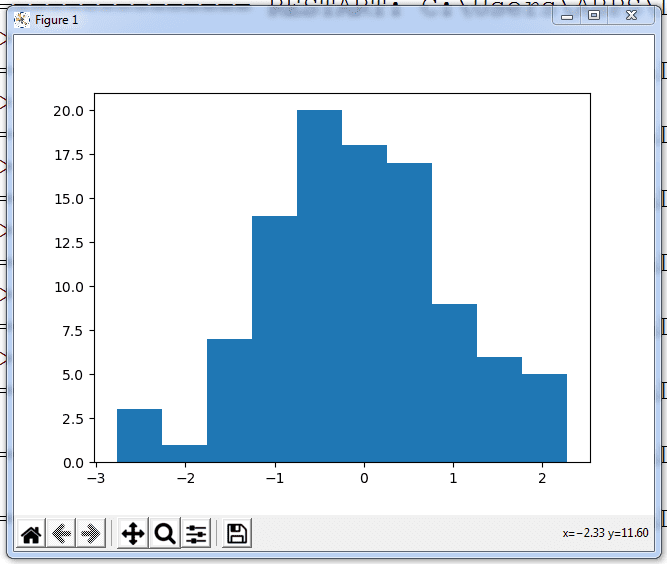In this article you will learn about Creating histogram with PyPlot. In previous article you learned about Creating Line chart and bar chart using Pyplot. The contents are as following:

Topics Covered

## What is histogram?

A histogram is quite similar to vertical bar graph with no space in between vertical bars. When you have data which has data points fall between a particular range, you can use histogram to visualize this data. It is helpful to display statistical data or data inserted in measurable quantities. For ex. Marks, scores, units etc. It was first introduced by Karl Pearson.

## Creating Histogram

To create histogram in python hist() function is used. The syntax of hist() function is like this:

matplotlib.pyplot.hist(x, bins=value,cumulative=bool_val, histtype=type, align=alignment, orientation=orientation) where,

x: It is list to be plotted on histogram

bins: bins can be integer number computed with + 1 or generated by default.

cumulative: It is a boolean value i.e. either True or False. If provided True then bins are calculated where each bin gives the counts in that bin plus all bins for smaller values. The last bin gives total number of data points. The default value is false.

hisstype: It is an option parameter. It can be any one of these:

1. bar: Bar type histogram, it arranges data side by side if given data is multiple. It is by default histtype.
2. barstacked: When multiple data are stacked on top of each other
3. step: Generates a lineplot that is by default unfilled
4. stepfilled: Generates a lineplot that is by default filled

orientation: It can be used for bar – type histogram

Have let us take look at following codes:

```import matplotlib.pyplot as m
import numpy as np
x=np.random.randn(100)
m.hist(x)
m.show()```
Output
In above example, histogram is created for 100 random numbers. Rest all parameters are not provided, so it has taken default values. Now let us change the look of histogram.

## Changing the look of histogram

It can be provided with bins=n or directly the number next to x parameter. If you want to apply border colors for the bars you can use edgecolor parameter. To change the fill color of bars facecolor parameter is used. Observe the following code:
```import matplotlib.pyplot as m
import numpy as np
x=np.random.randn(100)
m.hist(x,20,edgecolor="blue",facecolor="r")
m.show()```
Output

## Using cumulative

Look at this code and output:
```import matplotlib.pyplot as m
import numpy as np
x=np.random.randn(100)
m.hist(x,20,cumulative= True, edgecolor="blue",facecolor="r")
m.show()```

Output

## Saving the histogram as image

You can use savefig() method to save the histogram. Consider above example and add this line into the program.
m.savefig(“histo.png”)
Open the python installation folder to view the saved image.
Examples:
1. Write python code to create histogram based on given data:
English: 77,66,88,99,55,44,33,79,68,83
Hindi: 56,89,70,50,60,65,90,80,47,82
```import matplotlib.pyplot as m
english=[77,66,88,99,55,44,33,79,68,83]
maths=[56,89,70,50,60,65,90,80,47,82]
m.hist([english,maths])
m.show()```
2.Write code to Create a horizontal histogram on above data.
```import matplotlib.pyplot as m
english=[77,66,88,99,55,44,33,79,68,83]
maths=[56,89,70,50,60,65,90,80,47,82]
m.hist([english,maths], orientation='horizontal')
m.show()```
3. Write code to change the horizontal histogram type to stepfilled and it should be cumulative.
```import matplotlib.pyplot as m
english=[77,66,88,99,55,44,33,79,68,83]
maths=[56,89,70,50,60,65,90,80,47,82]
m.hist([english,maths], orientation='horizontal',
histtype="stepfilled", cumulative=True)
m.show()```